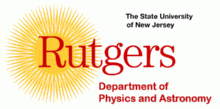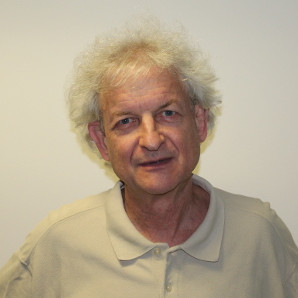# Daniel FriedanPosition: Faculty, NHETC (New High Energy Theory Center) Email address: dfriedan AT gmail.com Telephone: (848) 445-9062, secretary (848) 445-9050, fax (732) 445-4993 Office: Serin E366 Mailing address: Daniel Friedan Dept of Physics & Astronomy, Rutgers University 136 Frelinghuysen Road Piscataway, NJ 08854-8019 USA

teaching / research / papers / talks / other links

### Research

1. A theory of large distance physics  (1978 - )

In my 1980 phd thesis, I showed that the renormalization group flow of 2d quantum field theory produces the solutions to Einstein's equation for the gravitational metric of space-time. The coupling constants of the general 2d nonlinear field theory comprise a Riemannian metric on a manifold. The 2d renormalization group drives the Riemannian metric to a solution of Einstein's equation.

Soon after, this mechanism was partially incorporated into string theory. The 2d quantum field theory is the string world-sheet. The fixed point equation of the 2d rg — 2d scale invariance — is the consistency condition for the perturbative string S-matrix recipe. String theory provided more elaborate 2d nonlinear models whose couplings included space-time gauge and matter fields in addition to a Riemannian metric. The 2d couplings parametrize the string backgrounds. The 2d rg drives the string backgrounds to the classical solutions, the solutions of the classical space-time field equations.

Missing was a notion of a quantum string background related to a quantum field theory in space-time. There was no mechanism in string theory that produced quantum field theory — only a correspondence between the perturbative string S-matrix at low momenta and the perturbative S-matrix derived from the classical space-time field theory corresponding to the 2d rg fixed point.

In 2002, I proposed a mechanism that produces a space-time quantum field theory at large distances (in Planck units) and a quantum string background for string scattering at shorter distances, in such a way that they agree at the intermediate scale. This seems to me a perfectly acceptable form for a fundamental theory of physics to take. An S-matrix is not adequate as a complete theory of physics. Everything we know about the real physical world is described by quantum mechanics and its classical approximation. On the other hand, we have no reason to expect or need a quantum mechanical description of short distance physics, at distances many orders of magnitude smaller than we have any chance of observing experimentally. An S-matrix would be a quite suitable description of small distance physics.

The proposed mechanism is a 2d quantum field theory, the λ-model, a natural 2d nonlinear model whose target space is the space of classical space-time fields. The a priori measure of the 2d model is a functional integral on the classical fields — a quantum field theory in space-time. Perturbatively, this quantum field theory is the canonically quantized field theory. Semi-classical effects in the λ-model might produce non-canonical effects in the space-time quantum field theory. Winding modes would produce non-canonical degrees of freedom. Two-dimensional instantons would produce non-canonical couplings. Winding modes will be present in 4d space-time when there is an SU(2) gauge symmetry group. Two-dimensional instantons will be present when there is an SU(N) gauge symmetry, N ≥ 2. Predictions of non-canonical effects in the standard model might give a way to test the proposed mechanism.

2. Quantum field theories of extended objects/defects  (2016 - )

This is a project to construct a new class of quantum field theories of (n-1)-dimensional extended objects (defects) in d=2n space-time dimensions. For each ordinary 2d qft, there is to be a corresponding new qft of (n-1)-dimensional extended objects in any 2n-dimensional space-time manifold M. The quantum fields live on ``quasi Riemann surfaces'', which are certain complete metric spaces of integral (n-1)-currents in M. These quasi Riemann surfaces have analytic properties analogous to ordinary Riemann surfaces. The new qfts are to be constructed on the quasi Riemann surfaces just as ordinary 2d qfts are constructed on ordinary Riemann surfaces. The global symmetry group of the ordinary 2d qft becomes the gauge group of a local gauge symmetry in the new qft. I envision a wide expanse of new quantum field theory to explore.

3. Asymptotically large-scale quantum computers  (2005 - )

This is an attempt to formulate fundamental physical design principles for asymptotically large quantum computers and to characterize the physical systems that are suitable for asymptotically large quantum computers.

The basic argument is that near-critical physical systems are automatically protected by the renormalization group against almost all microscopic fluctuations. Only the fluctuations of the relevant and marginal couplings can affect the low energy quantum excitations. So near-critical systems are naturally suited for large-scale quantum computers. As a practical matter, large quantum computers in the real 3-dimensional world will be constructed as large-scale quantum circuits. Therefore, the proposal is that large-scale quantum computers should be constructed as near-critical quantum circuits in universality classes described by 1+1 dimensional quantum field theories.

The fundamental physical design principles should be based on the Kirchhoff laws for entropy flow in such near-critical quantum circuits, which are derived in the two papers listed above.

### More exposition

1. A theory of large distance physics  (1978 - )

2. Quantum field theories of extended objects/defects  (2016 - )

3. Asymptotically large-scale quantum computers  (2005 - )